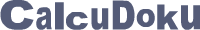﻿ CalcuDoku
 ВходРегистрация# CalcuDoku

## Заполните сетку цифрами, удовлетворяя указанным арифметическим действиям

• The math teaching puzzle from Japan
• Simple rules, very easy to learn
• Requires no language skills
• Wide range of logic and difficulty levels
• Develops logical deduction and reasoning

# SingleOp CalcuDoku

Еженедельно - новые головоломки

Each puzzle consists of a grid containing blocks surrounded by bold lines. The object is to fill all empty squares so that the numbers 1 to N (where N is the number of rows or columns in the grid) appear exactly once in each row and column and the numbers in each block produce the result shown in the top-left corner of the block according to the math operation appearing on the top of the grid. In CalcuDoku a number may be used more than once in the same block.

SingleOp CalcuDoku 4x4.a.n
Сложность: супер легкая
SingleOp CalcuDoku 5x5.m.n
Сложность: очень легкая
SingleOp CalcuDoku 6x6.a.n
Сложность: средняя

# DualOp CalcuDoku

Еженедельно - новые головоломки

Each puzzle consists of a grid containing blocks surrounded by bold lines. The object is to fill all empty squares so that the numbers 1 to N (where N is the number of rows or columns in the grid) appear exactly once in each row and column and the numbers in each block produce the result of the math operation shown in the top-left corner of the block. In CalcuDoku a number may be used more than once in the same block.

DualOp CalcuDoku 4x4.as.a
Сложность: супер легкая
DualOp CalcuDoku 5x5.as.a
Сложность: легкая
DualOp CalcuDoku 6x6.md.a
Сложность: средняя